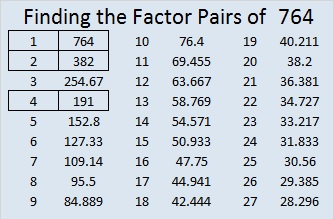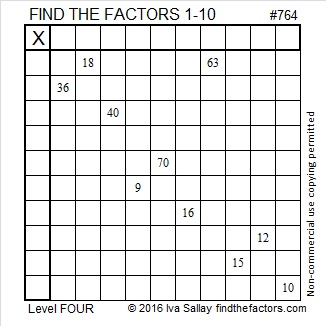# 764 and Level 4

• 764 is a composite number.
• Prime factorization: 764 = 2 x 2 x 191, which can be written 764 = (2^2) x 191
• The exponents in the prime factorization are 2 and 1. Adding one to each and multiplying we get (2 + 1)(1 + 1) = 3 x 2  = 6. Therefore 764 has exactly 6 factors.
• Factors of 764: 1, 2, 4, 191, 382, 764
• Factor pairs: 764 = 1 x 764, 2 x 382, or 4 x 191
• Taking the factor pair with the largest square number factor, we get √764 = (√4)(√191) = 2√191 ≈ 27.6405499.Here’s today’s puzzle:Print the puzzles or type the solution on this excel file: 10 Factors 2016-02-04

—————————————

Every number that ends in 4 is divisible by 2.

Every number that ends in 64 is divisible by 4.

Every number that ends in 764 is NOT divisible by 8, but every number ending in 764 can be written as the sum of 8 consecutive numbers.

For example, 92 + 93 + 94 + 95 + 96 + 97 + 98 + 99 = 764.

The first four digits of √764  are 27.64.

Wikipedia tells us that 764 is one of only two 3-digit “telephone numbers“. (911 is NOT the other one.)

—————————————This site uses Akismet to reduce spam. Learn how your comment data is processed.